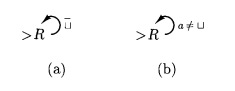# Turing Machine

## 图灵机定义

### 图灵机的运行规则

• 图灵机由 有穷状态控制器 组成，两者之间通过带头进行操作
• 控制器在每步完成两个功能
1. 让控制器进入新状态（和 DFA / NFA 类似）
2. 在当前扫描的带方格中 填入 一个符号 / 把读写头向左或向右 移动 一个带方格

### 图灵机的符号标示

A Turing Machine is 5-tuple $M=\left(K,\mathrm{\Sigma },\delta ,s,H\right)$

• $K$: a finite set of state
• $\mathrm{\Sigma }$: an alphabet (containing $▹$ and $\bigsqcup$)
• $s$: initial state
• $H\subseteq K$: a set of halting state
• $\delta$: transition function
• $\left(K-H\right)×\mathrm{\Sigma }\to K×\left(\mathrm{\Sigma }\cup \left\{←,\to \right\}\right)$
• $K-H$ is current state
• $\mathrm{\Sigma }$ is symbols
• $K$ is next state
• $\left(\mathrm{\Sigma }\cup \left\{←,\to \right\}\right)$ is writing or moving
• it satisfying
• for any $q\in K-H$, $\delta \left(q,▹\right)=\left(p,\to \right)$ for some $p\in K$
• for any $q\in K-H$, any $a\in \mathrm{\Sigma }$, if $\delta \left(q,a\right)=\left(p,b\right)$, $b\ne ▹$

• 带内容的简化记号 $\underset{―}{}$$w\underset{―}{a}u$ 表示 $\left(q,wa,u\right)$ 里的带内容

A configuration is a member of $K×▹\left(\mathrm{\Sigma }-\left\{▹\right\}{\right)}^{\ast }×\left(\left(\mathrm{\Sigma }-\left\{▹\right\}{\right)}^{\ast }\left(\mathrm{\Sigma }-\left\{▹,\bigsqcup \right\}\right)\cup \left\{e\right\}\right)$, and we say $\left({q}_{1},▹{w}_{1}{a}_{1}{u}_{1}\right){⊢}_{M}\left({q}_{2},▹{w}_{2}{a}_{2}{u}_{2}\right)$ if

1. writing: $\delta \left({q}_{1},{a}_{1}\right)=\left({q}_{2},{a}_{2}\right)$, ${a}_{2}\in \mathrm{\Sigma }-\left\{▹\right\}$, ${w}_{2}={w}_{1}$, ${u}_{2}={u}_{1}$
2. moving left: $\delta \left({q}_{1},{a}_{1}\right)=\left({q}_{2},←\right)$, ${w}_{1}={w}_{2}{a}_{2}$ and ${u}_{2}={a}_{1}{u}_{1}$
3. moving right: $\delta \left({q}_{1},a\right)=\left({q}_{2},\to \right)$, ${w}_{2}={w}_{1}{a}_{1}$, ${u}_{1}={a}_{2}{u}_{2}$
Similarly, we have the $\left({q}_{1},▹{w}_{1}{a}_{1}{u}_{1}\right){⊢}_{M}^{\ast }\left({q}_{2},▹{w}_{2}{a}_{2}{u}_{2}\right)$.

1. formal definition $M=\left(K,\mathrm{\Sigma },\delta ,s,H\right)$
2. Implemental-level description(diagram)
3. high-level description "pseudo code"

## 图灵机的记号

• 基本机器
• 写符号机 $a$：对每个 $b\in \mathrm{\Sigma }-\left\{▹\right\}$$\delta \left(s,b\right)=\left(h,a\right)$
• 移带头机 $L$, $R$
• 组合机器：直到上一台机器停机为止才应用从前一台机器到后一台机器的连接
flowchart LR
M1 --a--> M2
M1 --b--> M3
• 更多机器
• $R\to R$: can becomes simply $RR$, or even ${R}^{2}$
• ${R}_{\bigsqcup }$: 寻找当前扫描方格右方的第一个空格方格
• ${L}_{\bigsqcup }$: 寻找当前扫描方格左方的第一个空格方格
• ${R}_{\bigsqcup ^{―}}$: 寻找当前扫描方格右方的第一个非空格方格
• ${L}_{\bigsqcup ^{―}}$: 寻找当前扫描方格左方的第一个非空格方格
• $C$: transforms $\bigsqcup _{―}w\bigsqcup$ into $\bigsqcup w\bigsqcup w\bigsqcup _{―}$, where $w$ contains no blanks.
• ${S}_{\to }$: $\bigsqcup w\bigsqcup _{―}$ into $\bigsqcup \bigsqcup w\bigsqcup _{―}$, where $w$ contains no blanks.
what is overline $\stackrel{―}{a}$

If $a\in \mathrm{\Sigma }$ is any symbol, we can sometimes eliminate multiple arrows and labels by using $\stackrel{―}{a}$ to mean "any symbol except a."## 图灵机的应用

### Recoganize Language 识别语言

Let $M=\left(K,{\mathrm{\Sigma }}_{0},\mathrm{\Sigma },\delta ,s,\left\{y,h\right\}\right)$ be a TM, we say $M$ decides a language $L\subseteq {\mathrm{\Sigma }}_{0}^{\ast }$ if

1. for every $w\in L$, $\left(s,▹\bigsqcup _{―}w\right){⊢}_{M}^{\ast }\left(y,\dots \right)$, $M$ accepts $w$
2. for every $w\in L$, $\left(s,▹\bigsqcup _{―}w\right){⊢}_{M}^{\ast }\left(n,\dots \right)$, $M$ rejects $w$

A language $L$ is recursive(decidable) if it is decided by some Turing Machine.

we can said that M semidecides L(M)

Theorem: If $L$ is recurisive, $L$ must be recursively enumerable

Theorem: If $L$ is recurisive, $\stackrel{―}{L}$ must be recursive

graph
s(semidecide) --> re(recursively enumerable)
re --> s
d(decide) --> rl(recurive language)
rl --> d
rl --充分条件--> re
subgraph 决定
s
d
end

### Compute function 表示函数

Let $M=\left(K,\mathrm{\Sigma },\delta ,s,\left\{h\right\}\right)$ be a Turing machine, let ${\mathrm{\Sigma }}_{0}\subseteq \mathrm{\Sigma }-\left\{\bigsqcup ,▹\right\}$ be an alphabet, and let $w\in {\mathrm{\Sigma }}_{0}^{\ast }$. Suppose that M halts on input $w$, and that $\left(s,▹\bigsqcup _{―}w\right){⊢}_{M}^{\ast }\left(h,▹\bigsqcup _{―}y\right)$ for some $y\in {\mathrm{\Sigma }}_{0}^{\ast }$. Then $y$ is called the output of $M$ on input $w$, and is denoted $M\left(w\right)$.
Now let $f$ be any function from ${\mathrm{\Sigma }}_{0}^{\ast }$ to ${\mathrm{\Sigma }}_{0}^{\ast }$. We say that $M$ computes function $f$ if, for all $w\in {\mathrm{\Sigma }}_{0}^{\ast }$, $M\left(w\right)=f\left(w\right)$. A function $f$ is called recursive, if there is a Turing machine $M$ that computes $f$.

• 证明 recursive
1. 定义法：构造对应的 TM
2. 归约法：$A\le$ known recursive language
• 证明 recursively enumerable
1. 定义法：构造对应的 TM
2. 归约法：$A\le$ known recursively enumerable language
• 证明 non-recursive
1. 归约法：known recursively enumerable language $\le A$
• 证明 not recursively enumerable
1. If $A$ and $\overline{A}$ is recursively enumerable, then $A$ is recursive
2. 归约法：known not recursively enumerable language $\le A$

## 拓展图灵机

• multiple tapes
• two-way infinite tape
• two-dimensional tape
• random access
• non-determinstic turing machine
Non-determinstic Turing Machine

a non-deterministic Turing Machine (NTM) is 5-tuple $\left(K,\mathrm{\Sigma },\mathrm{\Delta },s,H\right)$

A NTM $M$ with input alphabet ${\mathrm{\Sigma }}_{0}$ semidecides $L\subseteq {\mathrm{\Sigma }}_{0}^{\ast }$ if for any $w\in {\mathrm{\Sigma }}_{0}^{\ast }$

• $w\in L$ iff $\left(s,▹\bigsqcup _{―}w\right){⊢}_{M}^{\ast }\left(h,\dots \right)$
• if $w\in L$ some branch halts
• if $w\notin L$ no branch halts

A NTM $M=\left(K,{\mathrm{\Sigma }}_{0},\mathrm{\Sigma },\mathrm{\Delta },s,\left\{y,n\right\}\right)$ with input alphabet ${\mathrm{\Sigma }}_{0}$ decides a language $L\subseteq {\mathrm{\Sigma }}_{0}^{\ast }$ if

1. there is a natrual number $N$, depending on $M$ and $w$ such that there is no configuration $C$ satisfying $\left(s,▹\bigsqcup _{―}w\right){⊢}_{M}^{N}C$
2. $w\in L$ iff $\left(s,▹\bigsqcup _{―}w\right){⊢}_{M}^{\ast }\left(y,\dots \right)$
Theorem: A NTM can be simulated by a DTM